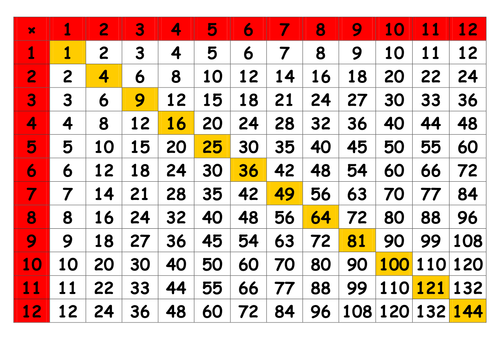# Definition of Multiplication Tables

A multiplication table is a table that shows you all the results of multiplying two of the integers from $1$ to $12$.

One number is taken from a row, and the other is taken from the column. The product is found in the square where the row and column overlap.

For example, the two times multiplication table includes: $2 \times 1 = 2$, $2 \times 2 = 4$, $2 \times 3 = 6$ and so on.

Other terms for Multiplication Tables include "Times Tables" and, simply, "Tables"### Description

The aim of this dictionary is to provide definitions to common mathematical terms. Students learn a new math skill every week at school, sometimes just before they start a new skill, if they want to look at what a specific term means, this is where this dictionary will become handy and a go-to guide for a student.

### Audience

Year 1 to Year 12 students

### Learning Objectives

Learn common math terms starting with letter M

Author: Subject Coach
You must be logged in as Student to ask a Question.